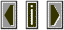FoldoverWhen signals in a digital system exceed the Nyquist frequency (i.e. half the sampling rate of the system) then the signal is said to foldover or reappear at a new frequency which is equal to the signal minus the difference between it and the sampling rate.   for example if one tries to generate a 13,000 Hz. signal when the sampling rate is 11,000 HZ then the signal will be folded over to 9,000 HZ. (11,000 - 2,000) see aliasing for sound example